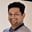Related Tags

java
communitycreator

# How to find the maximum subarray sum using the greedy approachTarun Telang

## Problem

### Description

Given an array of integers, find the sum of a subarraya contiguous subset of an array that contains at least one element and has the maximum sum.

### Example

In the array [-3, 1, -2, 5, 0, 3, 2, -4, 6], we can see that the subarray [5, 0, 3, 2, -4, 6] has a maximum sum of 12, so the output of this program should be 12.

## Algorithm

1. Iterate through the array

2. Keep track of the current sum and maximum sum encountered so far.

3. If current sum becomes negative:

• Ignore the subarray so far as it can in no way contribute to the maximum sum.
• Reset current sum to zero.
1. Otherwise, continue iterating the array until the end of the array.

2. After you have iterated through the entire array, return maximum sum.

## Code

class Solution {

public static int maxSubArray(int[] nums) {
int currentSum = 0; // current sum
int maximumSum = Integer.MIN_VALUE; // maximum sum to be returned
for(int i = 0; i < nums.length; i++)
{
currentSum = currentSum + nums[i];

if (currentSum > maximumSum)
maximumSum = currentSum;

// if currentSum is negative ignore
// and consider a new subarray
if (currentSum < 0)
currentSum = 0;
}
return maximumSum;
}

public static void main(String[] args) {
int array[] = new int[] {-3, 1, -2, 5, 0, 3, 2, -4, 6};
int max = maxSubArray(array);
System.out.println(max);
}
}
Code to find the maximum subarray sum

## Conclusion

The above program uses a greedy approach to continuously maximize the current sum.

Since we are only looping through the array once, the algorithm’s time complexity is $O(n)$, where $n$ is the length of the array.

The space complexity of this algorithm is $O(1)$, as we don’t need any additional memory (other than variables to store currentSum and maximumSum).

RELATED TAGS

java
communitycreator

CONTRIBUTORTarun Telang
RELATED COURSES

View all Courses

Keep Exploring

Learn in-demand tech skills in half the time